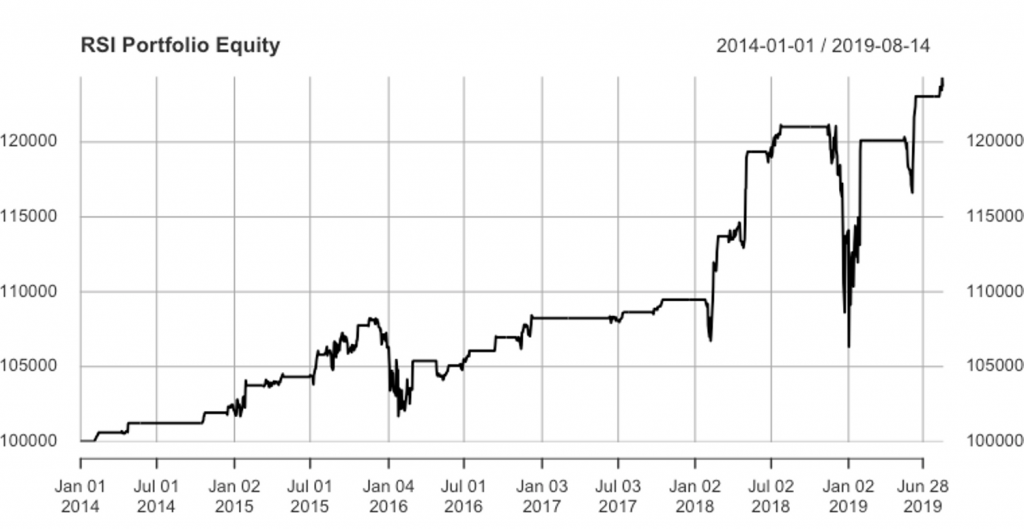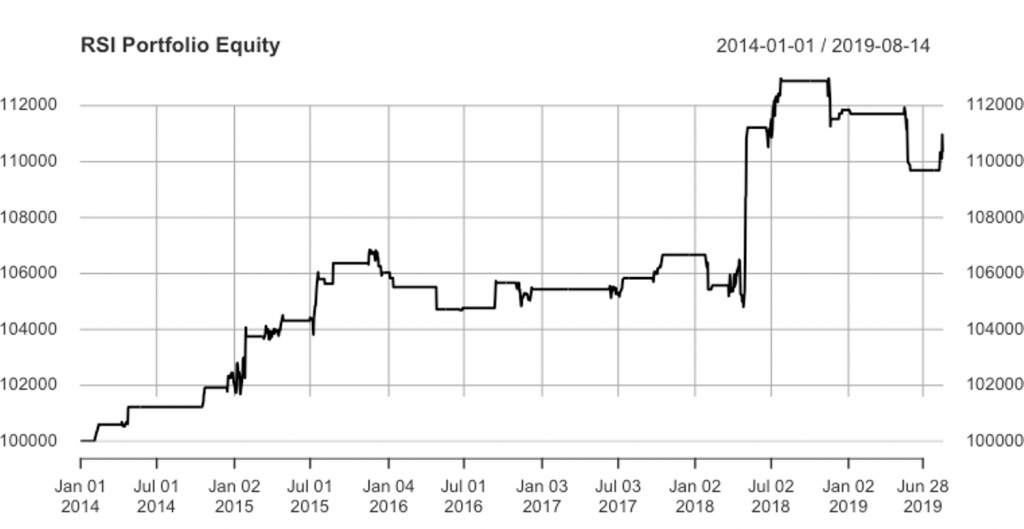# Quantstrat Example in R - RSI Strategy

In this Quantstrat case study, we will create a strategy with the Relative Strength Index (RSI) indicator that gives signals related to overbought and oversold regimes.

### RSI Strategy Entry and Exit Signals

In our strategy, we will work with the RSI signal to generate long positions only. We will analyze the strategy with 2 different exit conditions during the whole period.

The first exit condition is when the RSI is greater than 70. In this case we liquidate our long position by selling the asset. This exit point has good effect on some occasions but has the problem that doesn’t prevent for a big fall in prices that could cause high drawdowns on the strategy.

The second exit condition is to add a stop loss. Using a stop loss is more conservative because the price could reach the stop loss and then starts to rise again, but we make sure that our capital will not go down below a certain level defined by the stop loss.

The next step consists of coding the strategy with quantstrat package with the first exit point (RSI threshold exit point), show the performance and risk metrics for this strategy and then run the same strategy with the stop loss order and get the performance and risk metrics. Finally we will compare both results.

Start a new R session and ensure that the quantstrat and ggplot2 libraries are loaded.

### Initialize Currency and Instruments

• Initialize current (USD)
• Create symbol variable (we will use the Apple stock - AAPL)
• Set timezone
• Define the stock metadata using the stock() function
currency('USD')

symbol <- 'AAPL'

Sys.setenv(TZ="UTC")

stock(symbol,currency='USD',multiplier=1)



### Define Dates and Load Historical Data

# The initDate is used in the initialization of the portfolio and orders objects, and should be less than the startDate, which is the date of the data.

initDate <- '2012-01-10'

startDate <- '2014-01-01'

startEquity <- 100000

endDate <- '2019-08-14'

# Fetch the data from tiingo api. This command require an api key that can be acquired in the tiingo site

# Get your free Tiingo API Token from https://api.tiingo.com/



### Initialize Portfolio, Account and Order Objects

# Name the strategy, portfolio and account with the same name

portName <- stratName <- acctName <- 'AAPLPort'

# Remove objects associated with a strategy

rm.strat(stratName)

# Initialization of the Portfolio, Account and Orders objects with their required parameters. The startEquity value in the initEq parameter on the account initialization is added in order to compute returns.

initPortf(portName,symbols=symbol,initDate = startDate)

initAcct(acctName,portfolios = portName,initDate = startDate,initEq = startEquity)

initOrders(portfolio=portName,startDate=startDate)


### Initialize and Store the Strategy

# Initialize and store the strategy

strategy(stratName,store=TRUE)


# Define RSI Indicator

add.indicator(strategy=stratName, name="RSI", arguments=list(price = quote(Cl(mktdata)), maType="EMA"), label="RSI")

# The applyIndicators function allow to observe the indicators for a specific symbol while making progress in building the strategy

test <- applyIndicators(stratName, mktdata=OHLC(AAPL))

tail(test,10)

AAPL.Open AAPL.High AAPL.Low AAPL.Close  rsi.RSI
2019-08-01  213.0837  217.1979 205.9545   207.6345 53.73663
2019-08-02  204.7456  205.6422 200.8605   203.2414 41.63635
2019-08-05  197.2344  197.8909 191.8450   192.6021 25.55593
2019-08-06  195.5608  197.3111 193.2995   196.2482 35.41851
2019-08-07  194.6642  198.7984 193.0803   198.2804 40.48905
2019-08-08  199.4360  202.7532 198.6290   202.6536 50.19806
2019-08-09  201.3000  202.7600 199.2900   200.9900 46.84356
2019-08-12  199.6200  202.0516 199.1500   200.4800 45.76186
2019-08-13  201.0200  212.1400 200.8300   208.9700 62.42724
2019-08-14  203.1600  206.4400 202.5869   202.7500 49.55560


We will add the signals for entry and exit. The RSI signal to generate long positions when RSI crosses 30, and the exit signal when reaches 70.

add.signal(strategy = stratName, name="sigThreshold",
arguments = list(threshold=30,       column="RSI",relationship="lt",cross =TRUE),label="LongEntry")

arguments = list(threshold=70, column="RSI",relationship="gt",cross =TRUE),label="LongExit")

# With applySignal(), it is possible to observe the signal columns as well as the remaining information of the mktdata object.

sig <- applySignals(stratName,mktdata)

AAPL.Open AAPL.High AAPL.Low AAPL.Close  rsi.RSI LongEntry LongExit
2019-08-01  213.0837  217.1979 205.9545   207.6345 53.73663         0        0
2019-08-02  204.7456  205.6422 200.8605   203.2414 41.63635         0        0
2019-08-05  197.2344  197.8909 191.8450   192.6021 25.55593         1        0
2019-08-06  195.5608  197.3111 193.2995   196.2482 35.41851         0        0
2019-08-07  194.6642  198.7984 193.0803   198.2804 40.48905         0        0
2019-08-08  199.4360  202.7532 198.6290   202.6536 50.19806         0        0
2019-08-09  201.3000  202.7600 199.2900   200.9900 46.84356         0        0
2019-08-12  199.6200  202.0516 199.1500   200.4800 45.76186         0        0
2019-08-13  201.0200  212.1400 200.8300   208.9700 62.42724         0        0
2019-08-14  203.1600  206.4400 202.5869   202.7500 49.55560         0        0



add.rule(strategy = stratName,name='ruleSignal',
arguments = list(sigcol="LongEntry",sigval=TRUE,
orderqty= 100,  ordertype="market", orderside="long",
replace=FALSE),
label="longEnter", enabled=TRUE,  type="enter")

arguments = list(sigcol="LongExit",sigval=TRUE,
orderqty= "all",  ordertype="market", orderside="long",
replace=FALSE),
label="longExit", enabled=TRUE,  type="exit")



### Apply the Strategy to the Portfolio

We will now apply the strategy to portfolio and then update the portfolio, account and equity.

# Apply strategy to the portfolio

t1 <- Sys.time()

results <- applyStrategy(stratName,portfolios = portName,symbols = symbol)

t2 <- Sys.time()

print(t2-t1)

# Set up analytics. Update portfolio, account and equity

updatePortf(portName)

dateRange <- time(getPortfolio(portName)$summary)[-1] updateAcct(portName,dateRange) updateEndEq(acctName)  ### Portfolio Equity Returns tStats <- tradeStats(Portfolios = portName, use="trades", inclZeroDays=FALSE) # Plot the equity curve of the strategy final_acct <- getAccount(acctName) final_acct options(width=70) plot(final_acct$summary$End.Eq, main = "RSI Portfolio Equity")  #### APPL Portfolio Equity returns – First RSI StrategyThe strategy has positive returns in the whole period. However we can observe a huge drawdown at the end of 2018. As we stated in the risk management section, the stop loss prevents high equity losses when market go down as is the case of AAPL stock at the end of 2018. Let’s see some performance and risk metrics of this strategy. ### Strategy Statistics tab.profit <- tStats %>% select(Net.Trading.PL, Gross.Profits, Gross.Losses, Profit.Factor) t(tab.profit) AAPL Net.Trading.PL 23699.883948 Gross.Profits 27293.575028 Gross.Losses -3593.691080 Profit.Factor 7.594858 tab.wins <- tstats %>% select(Avg.Trade.PL, Avg.Win.Trade, Avg.Losing.Trade, Avg.WinLoss.Ratio) t(tab.wins) AAPL Avg.Trade.PL 1184.994197 Avg.Win.Trade 1605.504413 Avg.Losing.Trade -1197.897027 Avg.WinLoss.Ratio 1.340269 # Note: The pipe operator ( %>% )to select specific columns in a part of the dplyr package.  ### Obtain Risk Metrics rets <- PortfReturns(Account = acctName) rownames(rets) <- NULL tab.perf <- table.Arbitrary(rets, metrics=c( "Return.cumulative", "Return.annualized", "SharpeRatio.annualized", "CalmarRatio"), metricsNames=c( "Cumulative Return", "Annualized Return", "Annualized Sharpe Ratio", "Calmar Ratio")) tab.perf AAPL.DailyEqPL Cumulative Return 0.24846722 Annualized Return 0.04034198 Annualized Sharpe Ratio 0.55102551 Calmar Ratio 0.28209635 tab.risk <- table.Arbitrary(rets, metrics=c( "StdDev.annualized", "maxDrawdown" ), metricsNames=c( "Annualized StdDev", "Max DrawDown")) tab.risk AAPL.DailyEqPL Annualized StdDev 0.07321255 Max DrawDown 0.14300782  The Maximum Drawdown is 14% of the Equity. This represents a big drawdown and in some circumstances is not acceptable. Some strategies are constrained to have a Maximum Drawdown lower than a threshold. For this reason is important to run the same strategy and observe the stop loss effect to prevent big losses. ### RSI Stop Loss Order Exit We will revise our strategy to add a stop loss signal. This is to prevent large drawdowns. ### Initialize Variables and Objects .orderqty <- 100 .txnfees <- -10 .stoploss <- 0.05 # Stop loss percentage (5%) applied to the fill price of the entry order symbols <- c('AAPL') # Name the strategy, portfolio and account with the same name portName <- stratName <- acctName <- 'AAPLPort' rm.strat(portName) rm.strat(acctName) # For stocks the multiplier is always 1 stock(symbols, currency="USD", multiplier=1) # Remove objects associated with a strategy rm.strat(stratName) # Initialization of the Portfolio, Account and Orders objects with their required parameters. The startEquity value in the initEq parameter on the account initialization is added in order to compute returns. initPortf(portName,symbols=symbol,initDate = startDate) initAcct(acctName,portfolios = portName,initDate = startDate,initEq = startEquity) initOrders(portfolio=portName,startDate=startDate) # Initialize and store the strategy strategy(stratName,store=TRUE)  ### Add Strategy Indicators # Define RSI Indicator add.indicator(strategy=stratName, name="RSI", arguments=list(price = quote(Cl(mktdata)), maType="EMA"), label="RSI")  ### Add Signals # Add Signals add.signal(strategy = stratName, name="sigThreshold", arguments = list(threshold=30, column="RSI",relationship="lt",cross =TRUE),label="LongEntry") add.signal(strategy = stratName, name="sigThreshold", arguments = list(threshold=70, column="RSI",relationship="gt",cross =TRUE),label="LongExit")  ### Add Strategy Rules add.rule(strategy = stratName,name='ruleSignal', arguments = list(sigcol="LongEntry",sigval=TRUE, orderqty= 100, ordertype="market", orderside="long", replace=FALSE), label="longEnter", enabled=TRUE, type="enter") add.rule(strategy = stratName,name='ruleSignal', arguments = list(sigcol="LongExit",sigval=TRUE, orderqty= "all", ordertype="market", orderside="long", replace=FALSE), label="longExit", enabled=TRUE, type="exit") # This third rule adds the stop loss condition add.rule(stratName, name = "ruleSignal", arguments = list(sigcol = "LongEntry" , sigval = TRUE, replace = FALSE, orderside = "long", ordertype = "stoplimit", tmult = TRUE, threshold = quote(.stoploss), TxnFees = -10, orderqty = "all"), type = "chain", parent = "longEnter", label = "StopLossLONG", enabled = FALSE)  With these rules, the exit points can be triggered in two situations. First when the RSI reach the 70 threshold while we are in the market, and secondly when the AAPL price reached the defined stop loss of 5% of the price. The rule for the stop loss need a threshold parameter with the percentage of the filled price that the stop loss represents, and we should add the “chain” value to the type parameter, and invoke the parent order of the stop loss order which is the longEnter order. for(symbol in symbols){ addPosLimit(portfolio = portName, symbol = symbol, timestamp = startDate, maxpos = .orderqty) }  We have defined the stop loss order with the enable parameter set to FALSE, so here we enable the stop loss order: enable.rule(stratName, type = "chain", label = "StopLoss")  ### Apply Strategy #apply strategy t1 <- Sys.time() results <- applyStrategy(stratName,portfolios = portName,symbols = symbols) t2 <- Sys.time() print(t2-t1)  ### Update Portfolio, Account and Equity #set up analytics updatePortf(portName) dateRange <- time(getPortfolio(portName)$summary)[-1]

updateAcct(portName,dateRange)

updateEndEq(acctName)


### New Performance Metrics

tStats <- tradeStats(Portfolios = portName, use="trades", inclZeroDays=FALSE)

final_acct <- getAccount(acctName)

options(width=70)

plot(final_acct$summary$End.Eq, main = "RSI Portfolio Equity")


#### APPL Portfolio Equity returns – Second RSI StrategyThe first impression is while this second strategy has less returns at the end of the period compared to the first strategy, in this case we prevent the strategy from large drawdowns. With the stop loss parametrization we have avoided big losses.

# Performance and Risk Metrics
# Profits
tab.profit <- tStats %>%
t(tab.profit)
AAPL
Gross.Profits  18134.854581
Gross.Losses   -7793.977272
Profit.Factor      2.326778

tab.wins <- tStats %>%
t(tab.wins)

AAPL
Avg.WinLoss.Ratio    1.628745

rets <- PortfReturns(Account = acctName)
rownames(rets) <- NULL

tab.perf <- table.Arbitrary(rets,
metrics=c(
"Return.cumulative",
"Return.annualized",
"SharpeRatio.annualized",
"CalmarRatio"),
metricsNames=c(
"Cumulative Return",
"Annualized Return",
"Annualized Sharpe Ratio",
"Calmar Ratio"))
tab.perf

AAPL.DailyEqPL
Cumulative Return           0.10713135
Annualized Return           0.01830312
Annualized Sharpe Ratio     0.75692880
Calmar Ratio                0.55756178


### Risk Statistics

# Risk Statistics

tab.risk <- table.Arbitrary(rets,
metrics=c(
"StdDev.annualized",
"maxDrawdown"),
metricsNames=c(
"Annualized StdDev",
"Max DrawDown"))
tab.risk

AAPL.DailyEqPL
Annualized StdDev     0.02418077
Max DrawDown          0.03282708


The Max Drawdown when we set the stop loss in the RSI strategy is 3.28% while in the first RSI strategy (without stop loss), the Max Drawdown was 14%. The RSI strategy without the Stop Loss shows better returns at the end of the period, but when we add a stop loss, we prevent from large losses when market drops and both the Sharpe Ratio and Calmar Ratio improves. To conclude, if our decision about strategy selection, is based on the Sharpe Ratio (like many strategies), we should select the RSI with the stop loss order as it has a higher Sharpe Ratio than the RSI strategy without the stop loss.

Get our Data Science for Finance Bundle for just $29$51.